图像处理中的中值滤波_均值滤波、中值滤波、高斯滤波 图像处理函数的适用图像 - CSDN

• 原理：模板中心对准待处理像素，对模板下的对应像素进行灰度值排序，将中值赋给当前像素 Matlab代码： clear,clc; car = imread('sport car.pgm'); noise_car = imnoise(car,'salt &amp; pepper',0.02); [h,w...
原理：模板中心对准待处理像素，对模板下的对应像素进行灰度值排序，将中值赋给当前像素

Matlab代码：

clear,clc;
noise_car = imnoise(car,'salt & pepper',0.02);
[h,w] = size(car);
new_car1 = zeros(h+2,w+2);
new_car1(2:h+1,2:w+1) = noise_car;
copy_car = double(new_car1);
% 滑动窗口，进行中值滤波
for i = 2:h+1
for j = 2:w+1
end
end
new_car1 = new_car1(2:h+1,2:w+1);
new_car1 = uint8(new_car1);
new_car2 = medfilt2(noise_car,[3,3]);   % 调用medfilt2()函数

figure;
subplot(121),imshow(car),title('原图像');
subplot(122),imshow(noise_car),title('椒盐噪声图像');
figure;
subplot(121),imshow(new_car1),title('中值滤波图像');
subplot(122),imshow(new_car2),title('中值滤波图像（medfilt2）')

运行结果：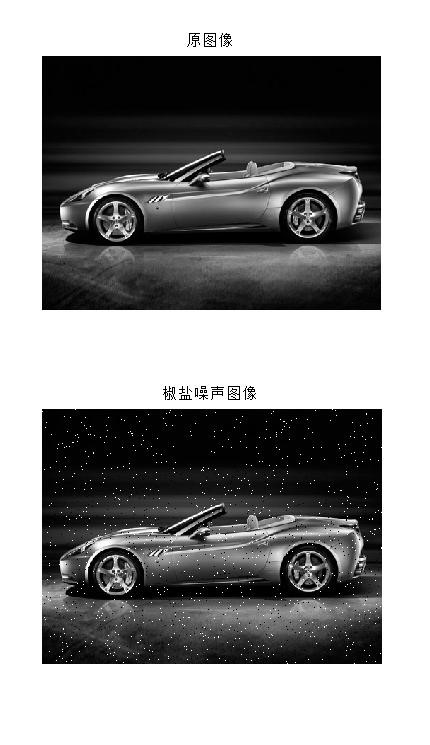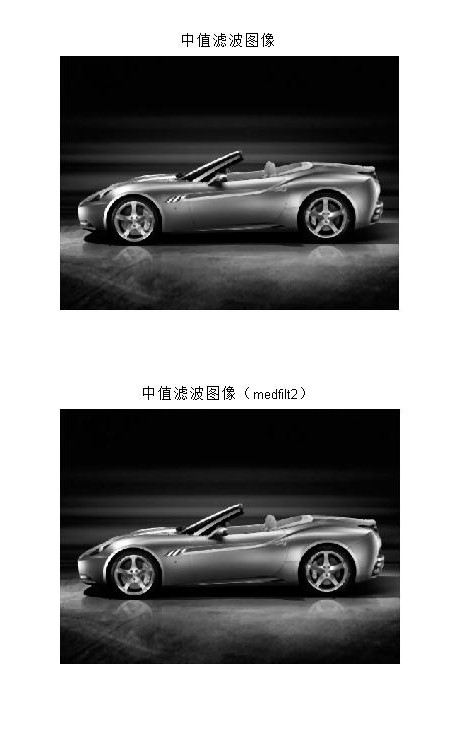展开全文• import numpy as np import scipy.ndimage import cv2 def convert_2d(r): n = 10 s = scipy.ndimage.median_filter(r, (n, n)) return s.astype(np.uint8) def convert_3d(r): s_dsplit = [] ...
中值滤波器将其像素邻域内的灰度中值代替代替该像素的值。中值滤波器的使用非常普遍, 这是因为对于一定类型的随机噪声, 它提供了一种优秀的去噪能力, 比小尺寸的均值滤波器模糊程度明显要低。中值滤波器对处理脉冲噪声(也称椒盐噪声)非常有效, 因为该噪声是以黑白点叠加在图像上面的。
import numpy as np
import scipy.ndimage
import cv2

def convert_2d(r):
n = 10
s = scipy.ndimage.median_filter(r, (n, n))
return s.astype(np.uint8)

def convert_3d(r):
s_dsplit = []
for d in range(r.shape):
rr = r[:, :, d]
ss = convert_2d(rr)
s_dsplit.append(ss)
s = np.dstack(s_dsplit)
return s

im_convert_mat = convert_3d(im)
cv2.imshow('median', im_convert_mat)
cv2.imwrite('median.jpg', im_convert_mat)
cv2.waitKey()展开全文数字图像处理 中值滤波
• 中值滤波的基本原理是把数字图像或数字序列一点的值用该点的一个邻域各点值的中值代替，让周围的像素值接近的真实值，从而消除孤立的噪声点。方法是用某种结构的二维滑动模板，将板内像素按照像素值的大小进行...
1 中值滤波概述
中值滤波是基于排序统计理论的一种能有效抑制噪声的非线性信号平滑处理技术，它将每一像素点的灰度值设置为该点某邻域窗口内的所有像素点灰度值的中值。
中值滤波的基本原理是把数字图像或数字序列中一点的值用该点的一个邻域中各点值的中值代替，让周围的像素值接近的真实值，从而消除孤立的噪声点。方法是用某种结构的二维滑动模板，将板内像素按照像素值的大小进行排序，生成单调上升（或下降）的为二维数据序列。二维中值滤波输出为g（x,y）=med{f(x-k,y-l),(k,l∈W)} ，其中，f(x,y)，g(x,y)分别为原始图像和处理后图像。W为二维模板，通常为3*3，5*5区域，也可以是不同的的形状，如线状，圆形，十字形，圆环形等。
2 中值滤波算法原理
中值滤波数学实现：对一个数字信号序列xj(-∞<j<∞)进行滤波处理时，首先要定义一个长度为奇数的L长窗口，L=2N+1，N为正整数。设在某一个时刻，窗口内的信号样本为x(i-N)，…，x(i)，…，x(i+N)，其中x(i)为位于窗口中心的信号样本值。对这L个信号样本值按从小到大的顺序排列后，其中值，在i处的样值，便定义为中值滤波的输出值。
在实际应用中，随着所选用窗口长度的增加，滤波的计算量将会迅速增加。因此，寻求中值滤波的快速算法，是中值滤波理论的一个重要研究内容。中值滤波的快速算法，一般采用下述三种方式：①直方图数据修正法；②样本值二进制表示逻辑判断法；③数字和模拟的选择网络法。
对中值滤波的理论研究，还集中于统计特性分析和根序列的描述方面。当一个信号序列经一特定窗口长度的中值滤波反复处理后，它会收敛于某一个不再变化的序列，这个序列称为中值滤波的根序列。根序列是描述中值滤波特性的一个重要概念。通过对根序列结构的研究，可以确定原信号序列中，哪些成分可以经中值滤波后保留下来，哪些成分将被抑制。这对确定中值滤波器的窗口长度，提供了重要依据。用VLSI实现的中值滤波器芯片，可供实时处理中应用。
3 中值滤波算法流程4 中值滤波应用及优势
中值滤波法对消除椒盐噪声非常有效，在光学测量条纹图象的相位分析处理方法中有特殊作用，但在条纹中心分析方法中作用不大.
中值滤波在图像处理中,常用于保护边缘信息,是经典的平滑噪声的方法。
5 中值滤波代码实现1 // zhongzhilvbo.cpp : 定义控制台应用程序的入口点。
2 //
3
4 #include <stdio.h>
5 #include <math.h>
6 #include <memory.h>
7 #include <conio.h>
8 #include <stdlib.h>
9 #include <windows.h>
10 #include "stdafx.h"
11 //原图象的宽度和高度
12 #define width 352
13 #define higth 288
14
15 int lvbo(unsigned char D)
16 {
17     unsigned int temp;
18     int i,j;
19
20     for(i=0;i<9;i++)
21     {
22         for(j=0;j<9-i;j++)
23         {
24             if(D[i]>D[j+1])
25             {
26                 temp=D[i];
27                 D[i]=D[j+1];
28                 D[j+1]=temp;
29             }
30         }
31     }
32
33     return D;
34 }
35
36 void main()
37 {
38     FILE *fp,*newfp;
39     int i,j;
40
41     if(!(fp=fopen("fmh1.bmp","rb")))
42     {
43         printf("Open file %s error!\n","k.bmp");
44         return ;
45     }
46
47     if(!(newfp=fopen("fmout.bmp","wb")))
48     {
49         printf("Open file %s error!\n","result.bmp");
50         return ;
51     }
52
53     unsigned char buffer[54+1024];//定义原图像头缓冲区
55
56     unsigned long length=width*higth;//图像的总象素个数
58     unsigned char writeData[higth][width];               //用于存储原图数据的数组
59
61
62     for(i=0;i<higth;i++)
63     {
64         for(j=0;j<width;j++)
65         {
67         }
68     }
69
70     unsigned char D;        //定义选取框
71     for(i=1;i<higth-1;i++)
72     {
73         for(j=1;j<width-1;j++)
74         {
84             writeData[i][j]=lvbo(D);
85         }
86     }
87
88     fwrite(buffer,sizeof(unsigned char),54+1024,newfp);
89     fwrite(writeData,sizeof(unsigned char),length,newfp);
90     fclose(newfp);
91     fclose(fp);
92
93     return ;
94 }经过中值滤波处理后的对比图片：展开全文• ## 图像处理：中值滤波&均值滤波

万次阅读 多人点赞 2018-08-16 20:06:58
转自：openCV之滤波&amp;均值滤波（及代码实现）：https://blog.csdn.net/weixin_37720172/article/details/72627543  在开始我们今天的博客之前，我们需要先了解一下什么是滤波： openCV之滤波&...
转自：openCV之中值滤波&均值滤波（及代码实现）：https://blog.csdn.net/weixin_37720172/article/details/72627543

在开始我们今天的博客之前，我们需要先了解一下什么是滤波：

openCV之中值滤波&均值滤波（及代码实现）首先我们看一下图像滤波的概念。图像滤波，即在尽量保留图像细节特征的条件下对目标图像的噪声进行抑制，是图像预处理中不可缺少的操作，其处理效果的好坏将直接影响到后续图像处理和分析的有效性和可靠性。

下图左边是原图右边是噪声图：消除图像中的噪声成分叫作图像的平滑化或滤波操作。信号或图像的能量大部分集中在幅度谱的低频和中频段是很常见的，而在较高频段，感兴趣的信息经常被噪声淹没。因此一个能降低高频成分幅度的滤波器就能够减弱噪声的影响。
图像滤波的目的有两个:一是抽出对象的特征作为图像识别的特征模式;另一个是为适应图像处理的要求，消除图像数字化时所混入的噪声。
而对滤波处理的要求也有两条:一是不能损坏图像的轮廓及边缘等重要信息;二是使图像清晰视觉效果好。
平滑滤波是低频增强的空间域滤波技术。它的目的有两类：一类是模糊；另一类是消除噪音。
空间域的平滑滤波一般采用简单平均法进行，就是求邻近像元点的平均亮度值。邻域的大小与平滑的效果直接相关，邻域越大平滑的效果越好，但邻域过大，平滑会使边缘信息损失的越大，从而使输出的图像变得模糊，因此需合理选择邻域的大小。
关于滤波器，一种形象的比喻法是：我们可以把滤波器想象成一个包含加权系数的窗口，当使用这个滤波器平滑处理图像时，就把这个窗口放到图像之上，透过这个窗口来看我们得到的图像。

举一个滤波在我们生活中的应用：美颜的磨皮功能。如果将我们脸上坑坑洼洼比作是噪声的话，那么滤波算法就是来取出这些噪声，使我们自拍的皮肤看起来很光滑。

这篇博文会介绍中值滤波以及均值滤波两种算法

一.均值滤波

图片中一个方块区域（一般为3*3）内，中心点的像素为全部点像素值的平均值。均值滤波就是对于整张图片进行以上操作。

我们可以看下图的矩阵进行理解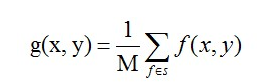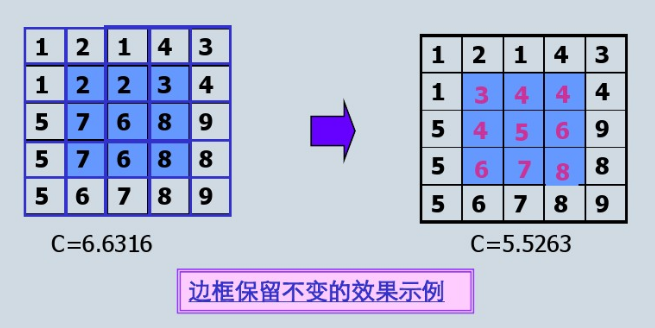缺陷：均值滤波本身存在着固有的缺陷，即它不能很好地保护图像细节，在图像去噪的同时也破坏了图像的细节部分，从而使图像变得模糊，不能很好地去除噪声点。特别是椒盐噪声

实现代码：

#include "opencv2/imgproc.hpp"
#include "opencv2/highgui.hpp"
#include<ctime>
using namespace cv;
using namespace std;

//均值滤波
void AverFiltering(const Mat &src,Mat &dst) {
if (!src.data) return;
//at访问像素点
for (int i = 1; i<src.rows; ++i)
for (int j = 1; j < src.cols; ++j) {
if ((i - 1 >= 0) && (j - 1) >= 0 && (i + 1)<src.rows && (j + 1)<src.cols) {//边缘不进行处理
dst.at<Vec3b>(i, j) = (src.at<Vec3b>(i, j) + src.at<Vec3b>(i - 1, j - 1) + src.at<Vec3b>(i - 1, j) + src.at<Vec3b>(i, j - 1) +
src.at<Vec3b>(i - 1, j + 1) + src.at<Vec3b>(i + 1, j - 1) + src.at<Vec3b>(i + 1, j + 1) + src.at<Vec3b>(i, j + 1) +
src.at<Vec3b>(i + 1, j)) / 9;
dst.at<Vec3b>(i, j) = (src.at<Vec3b>(i, j) + src.at<Vec3b>(i - 1, j - 1) + src.at<Vec3b>(i - 1, j) + src.at<Vec3b>(i, j - 1) +
src.at<Vec3b>(i - 1, j + 1) + src.at<Vec3b>(i + 1, j - 1) + src.at<Vec3b>(i + 1, j + 1) + src.at<Vec3b>(i, j + 1) +
src.at<Vec3b>(i + 1, j)) / 9;
dst.at<Vec3b>(i, j) = (src.at<Vec3b>(i, j) + src.at<Vec3b>(i - 1, j - 1) + src.at<Vec3b>(i - 1, j) + src.at<Vec3b>(i, j - 1) +
src.at<Vec3b>(i - 1, j + 1) + src.at<Vec3b>(i + 1, j - 1) + src.at<Vec3b>(i + 1, j + 1) + src.at<Vec3b>(i, j + 1) +
src.at<Vec3b>(i + 1, j)) / 9;
}
else {//边缘赋值
dst.at<Vec3b>(i, j) = src.at<Vec3b>(i, j);
dst.at<Vec3b>(i, j) = src.at<Vec3b>(i, j);
dst.at<Vec3b>(i, j) = src.at<Vec3b>(i, j);
}
}
}
//图像椒盐化
void salt(Mat &image, int num) {
if (!image.data) return;//防止传入空图
int i, j;
srand(time(NULL));
for (int x = 0; x < num; ++x) {
i = rand() % image.rows;
j = rand() % image.cols;
image.at<Vec3b>(i, j) = 255;
image.at<Vec3b>(i, j) = 255;
image.at<Vec3b>(i, j) = 255;
}
}
void main() {

Mat Salt_Image;
image.copyTo(Salt_Image);
salt(Salt_Image, 3000);

Mat image1(image.size(), image.type());
Mat image2;
AverFiltering(Salt_Image, image1);
blur(Salt_Image, image2, Size(3, 3));//openCV库自带的均值滤波函数
imshow("原图", image);
imshow("自定义均值滤波", image1);
imshow("openCV自带的均值滤波", image2);
waitKey();
}


效果图：可以看到图片变模糊而且噪声并没有很有效的去除，该算法只是模糊化了图片而已。

二.中值滤波

首先，我们复习中值。在一连串数字｛1，4，6，8，9｝中，数字6就是这串数字的中值。由此我们可以应用到图像处理中。依然我们在图像中去3*3的矩阵，里面有9个像素点，我们将9个像素进行排序，最后将这个矩阵的中心点赋值为这九个像素的中值。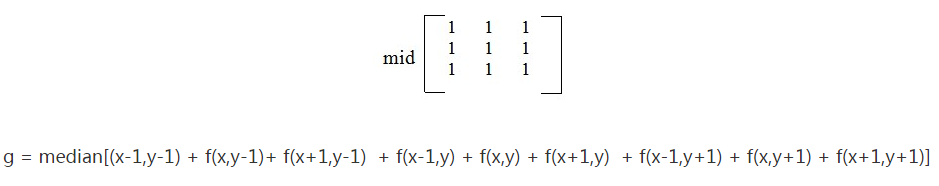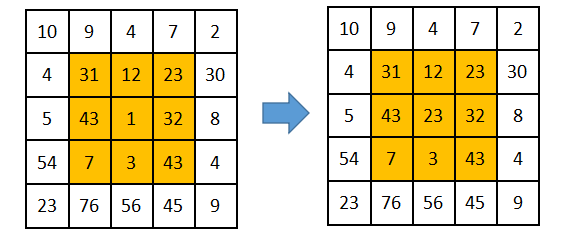代码：

//求九个数的中值
uchar Median(uchar n1, uchar n2, uchar n3, uchar n4, uchar n5,
uchar n6, uchar n7, uchar n8, uchar n9) {
uchar arr;
arr = n1;
arr = n2;
arr = n3;
arr = n4;
arr = n5;
arr = n6;
arr = n7;
arr = n8;
arr = n9;
for (int gap = 9 / 2; gap > 0; gap /= 2)//希尔排序
for (int i = gap; i < 9; ++i)
for (int j = i - gap; j >= 0 && arr[j] > arr[j + gap]; j -= gap)
swap(arr[j], arr[j + gap]);
return arr;//返回中值
}

//图像椒盐化
void salt(Mat &image, int num) {
if (!image.data) return;//防止传入空图
int i, j;
srand(time(NULL));
for (int x = 0; x < num; ++x) {
i = rand() % image.rows;
j = rand() % image.cols;
image.at<Vec3b>(i, j) = 255;
image.at<Vec3b>(i, j) = 255;
image.at<Vec3b>(i, j) = 255;
}
}

//中值滤波函数
void MedianFlitering(const Mat &src, Mat &dst) {
if (!src.data)return;
Mat _dst(src.size(), src.type());
for(int i=0;i<src.rows;++i)
for (int j=0; j < src.cols; ++j) {
if ((i - 1) > 0 && (i + 1) < src.rows && (j - 1) > 0 && (j + 1) < src.cols) {
_dst.at<Vec3b>(i, j) = Median(src.at<Vec3b>(i, j), src.at<Vec3b>(i + 1, j + 1),
src.at<Vec3b>(i + 1, j), src.at<Vec3b>(i, j + 1), src.at<Vec3b>(i + 1, j - 1),
src.at<Vec3b>(i - 1, j + 1), src.at<Vec3b>(i - 1, j), src.at<Vec3b>(i, j - 1),
src.at<Vec3b>(i - 1, j - 1));
_dst.at<Vec3b>(i, j) = Median(src.at<Vec3b>(i, j), src.at<Vec3b>(i + 1, j + 1),
src.at<Vec3b>(i + 1, j), src.at<Vec3b>(i, j + 1), src.at<Vec3b>(i + 1, j - 1),
src.at<Vec3b>(i - 1, j + 1), src.at<Vec3b>(i - 1, j), src.at<Vec3b>(i, j - 1),
src.at<Vec3b>(i - 1, j - 1));
_dst.at<Vec3b>(i, j) = Median(src.at<Vec3b>(i, j), src.at<Vec3b>(i + 1, j + 1),
src.at<Vec3b>(i + 1, j), src.at<Vec3b>(i, j + 1), src.at<Vec3b>(i + 1, j - 1),
src.at<Vec3b>(i - 1, j + 1), src.at<Vec3b>(i - 1, j), src.at<Vec3b>(i, j - 1),
src.at<Vec3b>(i - 1, j - 1));
}
else
_dst.at<Vec3b>(i, j) = src.at<Vec3b>(i, j);
}
_dst.copyTo(dst);//拷贝
}

void main() {

Mat Salt_Image;
image.copyTo(Salt_Image);
salt(Salt_Image, 3000);

Mat image3, image4;
MedianFlitering(Salt_Image, image3);
medianBlur(Salt_Image, image4, 3);
imshow("自定义中值滤波处理后", image3);
imshow("openCV自带的中值滤波", image4);
waitKey();
}


效果图：可以看到，椒盐噪声很好的被平滑了，而且也没均值那样模糊化太过于严重。

三 填充问题

在对图像应用滤波器进行过滤时，边界问题是一个需要处理的问题。一般来说，有3种处理的方法。

1. 不做边界处理

不对图像的边界作任何处理，在对图像进行滤波时，滤波器没有作用到图像的四周，因此图像的四周没有发生改变。

2. 填充0

对图像的边界做扩展，在扩展边界中填充0，对于边长为2k+1的方形滤波器，扩展的边界大小为k，若原来的图像为[m, n]，则扩展后图像变为[m+2k, n+2k]。进行滤波之后，图像会出现一条黑色的边框。

3. 填充最近像素值

扩展与 填充0 的扩展类似，只不过填充0的扩展是在扩展部分填充0，而这个方法是填充距离最近的像素的值。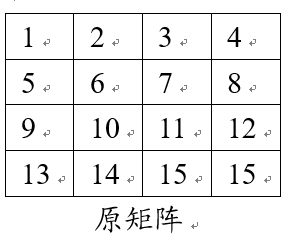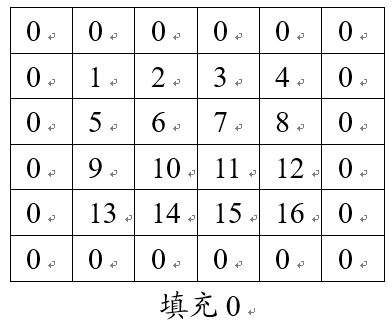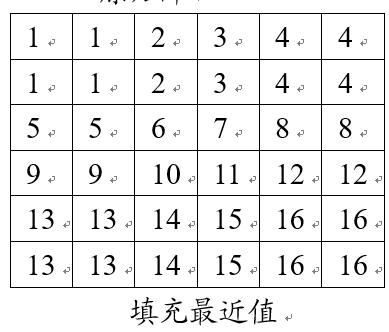四 总结：

均值滤波和和中值滤波都可以起到平滑图像，虑去噪声的功能。

均值滤波采用线性的方法，平均整个窗口范围内的像素值，均值滤波本身存在着固有的缺陷，即它不能很好地保护图像细节，在图像去噪的同时也破坏了图像的细节部分，从而使图像变得模糊，不能很好地去除噪声点。均值滤波对高斯噪声表现较好，对椒盐噪声表现较差。

中值滤波采用非线性的方法，它在平滑脉冲噪声方面非常有效,同时它可以保护图像尖锐的边缘，选择适当的点来替代污染点的值，所以处理效果好，对椒盐噪声表现较好，对高斯噪声表现较差。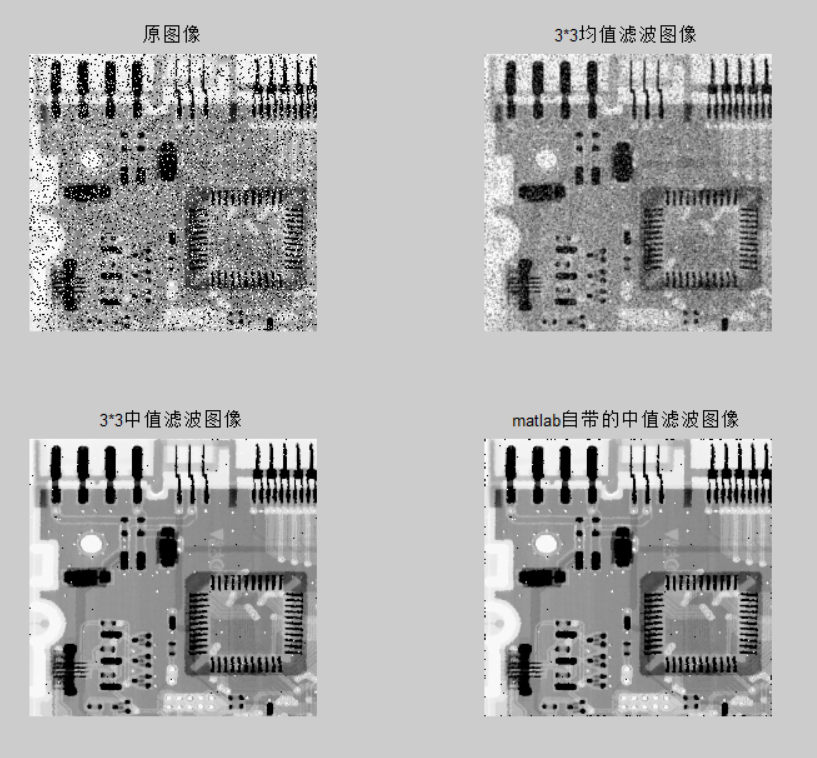参考：

1.均值滤波和中值滤波

https://blog.csdn.net/cjsh_123456/article/details/79261271

2.
展开全文图像处理 中值滤波 均值滤波
• 图像滤波：即在尽量保留图像细节特征的条件下对目标图像的噪声进行抑制，是图像预处理不可缺少的操作，其处理效果的好坏将直接影响到后续图像处理和分析的有效性和可靠性。 消除图像的噪声...图像平滑处理 中值滤波 图像处理 计算机视觉处理
• ## 中值滤波matlab

万次阅读 2016-04-07 09:17:00
摘 要 中值滤波技术是一种在去除噪声的同时能较好保护图像边缘细节的非线性技术，在图像增强和恢复等领域得到了广泛的应用...中值滤波图像处理，MATLAB 1. 引言 数字图像是建立在人眼的感光特性和大
• ## MATLAB的图像中值滤波

万次阅读 多人点赞 2018-11-28 09:58:22
下面来介绍一下图像中值滤波方法： 1、首先打开MATLAB软件，在其主界面的编辑器写入下列代码： I=imread('G:\MATLAB\bm.bmp'); %读取保存路径下的图片 I=rgb2gray(I); J=imnoise(I,'salt &amp; pepper',...MATLAB 中值滤波
• ## 中值滤波

万次阅读 2016-06-23 16:40:02
一、概述  数字图像的采样或传输在经过传感器或传输通道时经常受到噪声的干扰。为了便利进一步的图像操作，如：...但通常的中值滤波中的数据排序费时较多，特别在大窗口下，需要进行大量的数据比较工作，不利于图像
• ## [图像]中值滤波(Matlab实现)

万次阅读 多人点赞 2018-05-25 13:34:19
原理简述 中值滤波是基于排序统计理论的一种能有效抑制噪声的非线性信号处理技术。它也是一种邻域运算，类似于卷积，但是计算的不是加权求和，而是把数字图像或数字序列一点的值用该点的一个邻域各点值的中值...中值滤波 Matlab 图像处理
• 中值滤波就是将当前像素值替换为模板覆盖范围内的所有像素值大小居中那一个：对于一个3*3的模板，...为了验证上述结论，我先给一幅图像加入椒盐噪声，然后用分别用中值滤波、均值滤波处理图像，进行对比： #includeimage
• ## 中值滤波（matlab）

万次阅读 多人点赞 2019-02-16 16:42:00
中值滤波 中值滤波：是一种非线性数字滤波器技术， 用于降噪。 理解：去一个模板，可为3 * 3, 5 * 5,n * n…每次从图像中取出模板大小的矩阵，将所有元素排序，取中间值放入木板的中心位置，再还原到原图，...中值滤波 matlab 填充
• 中值滤波程序： clc; clear all; J=imread('F:\图像去噪\4.jpg'); I=rgb2gray(J); subplot(1,2,1); imshow(I); title('原始图像'); K=medfilt2(I,[4,4])%进行中值滤波; subplot(1,2,2); imshow(K); title('进行中值...Matlab 中值滤波
• 一般为方形邻域，也可以为圆形、十字形等等，然后将邻域各像素的灰度值排序，取其中间值作为中心像素灰度的新值，这里领域被称为窗口，当窗口移动时，利用中值滤波可以对图像进行平滑处理。其算法简单，时间复杂度...中值滤波
• ## 均值滤波和中值滤波

万次阅读 多人点赞 2018-02-05 20:19:58
均值滤波及中值滤波的区别 均值滤波和和中值滤波都可以起到平滑图像，虑去噪声的功能。均值滤波采用线性的方法，平均整个窗口范围内的像素值，均值滤波本身存在着固有的缺陷，即它不能很好地保护图像细节，在...matlab编程
• ## python 中值滤波

千次阅读 2017-07-21 12:50:49
中值滤波是数字信号处理和数字图像处理领域使用较多的预处理技术，使用邻域内所有信号的位数替换中心像素的值，可以在滤除异常值的情况下较好地保留纹理信息。该技术会在一定程度上造成图像模糊和失真，滤波窗口变...
• 椒盐噪声用中值滤波比较好 原因是：1.椒盐噪声是幅值近似相等但是随机的分布在不同...中值滤波缺点：对于点、线、尖顶的图像不适用中值滤波因为会出现自适应话。 高斯噪声用均值滤波比较好 原因是：1.高斯噪声的幅值近matlab 图片 噪声处理
• ## 自适应中值滤波及MATLAB实现

万次阅读 热门讨论 2016-12-30 10:39:17
自适应中值滤波器是以m*n的矩形窗口Sxy定义的滤波器区域内图像的...其matlab实现如下，并与中值滤波作比较。 clc; clear; f=imread('C:\Program Files\MATLAB\R2013a\bin\work\图像复原\Penguins.jpg'); image_gray=rgmatlab 图像处理 自适应中值滤波 噪声
• ## OpenCV—中值滤波

万次阅读 2018-06-27 16:44:44
与低通滤波不同的是，中值滤波有利于保留边缘的尖锐度，但它会洗去均匀介质区域的纹理。椒盐噪声椒盐噪声是由图像传感器，传输信道，解码处理等产生的黑白相间的亮暗点噪声。椒盐噪声是指两种噪声，一种是盐噪声...
• 均值滤波，是图像处理中最常用的手段，从频率域观点来看均值滤波是一种低通滤波器，高 频信号将会去掉，因此可以帮助消除图像尖锐噪声，实现图像平滑，模糊等功能。理想的均 值滤波是用每个像素和它周围像素计算...
• 盐噪声也称为脉冲噪声，是图像中经常见到的一种噪声，它是一种随机出现的白点或者黑点，可能是亮的区域有黑色像素或是在暗的区域有白色像素（或是两者皆有）。盐和胡椒噪声的成因可能是影像讯号受到突如其来的强烈...matlab 滤波 信号处理
...